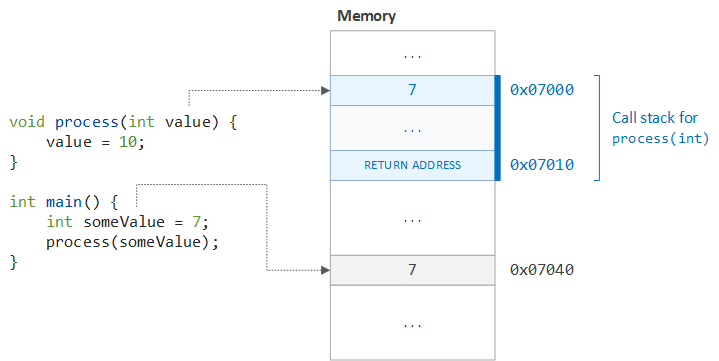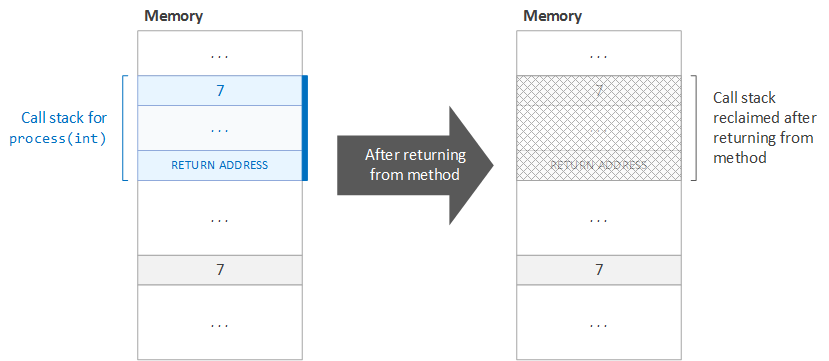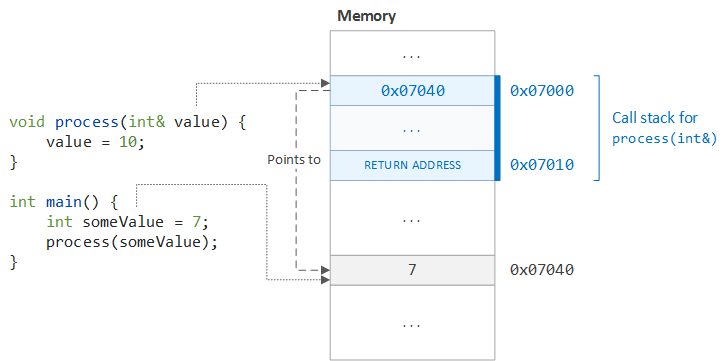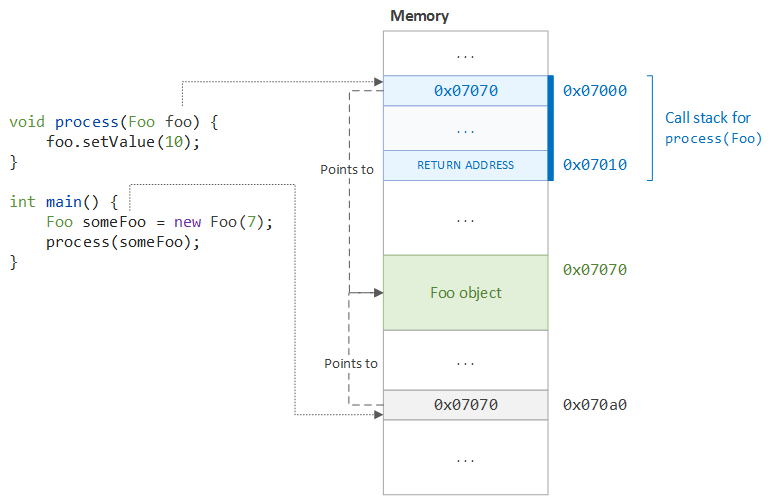{{announcement.body}}
{{announcement.title}}

# Passing by Value vs. Passing by Reference in Java

DZone 's Guide to

# Passing by Value vs. Passing by Reference in Java

### While many languages put information passing into the hands of developers, all data is passed by value in Java. See how to turn that restriction to your advantage.

· Java Zone ·
Free Resource

Comment (21)

Save
{{ articles.views | formatCount}} Views

Understanding the technique used to pass information between variables and into methods can be a difficult task for a Java developer, especially those accustomed to a much more verbose programming language, such as C or C++. In these expressive languages, the developer is solely responsible for determining the technique used to pass information between different parts of the system. For example, C++ allows a developer to explicitly pass a piece of data either by value, by reference, or by pointer. The compiler simply ensures that the selected technique is properly implemented and that no invalid operation is performed.

In the case of Java, these low-level details are abstracted, which both reduces the onus on the developer to select a proper means of passing data and increases the security of the language (by inhibiting the manipulation of pointers and directly addressing memory). In addition, though, this level of abstraction hides the details of the technique performed, which can obfuscate a developer's understanding of how data is passed in a program. In this article, we will examine the various techniques used to pass data and deep-dive into the technique that the Java Virtual Machine (JVM) and the Java Programming Language use to pass data, as well as explore some examples that demonstrate in practical terms what these techniques mean for a developer.

## Terminology

In general, there are two main techniques for passing data in a programming language: (1) passing by value and (2) passing by reference. While some languages consider passing by reference and passing by pointer two different techniques, in theory, one technique can be thought of as a specialization of the other, where a reference is simply an alias to an object, whose implementation is a pointer.

### Passing by Value

The first technique, passing by value, is defined as follows:

Passing by value constitutes copying of data, where changes to the copied value are not reflected in the original value

For example, if we call a method that accepts a single integer argument and the method makes an assignment to this argument, the assignment is not preserved once the method returns. While one might expect that the assignment is preserved after the method returns, the assignment is lost because the value placed on the call stack was a copy of the value passed into the method, as illustrated in the snippet below:

``````#include <iostream>

using namespace std;

void process(int value) {
cout << "Value passed into function: " << value << endl;
value = 10;
cout << "Value before leaving function: " << value << endl;
}

int main() {
int someValue = 7;
cout << "Value before function call: " << someValue << endl;
process(someValue);
cout << "Value after function call: " << someValue << endl;

return 0;
}``````

If we execute this code, we obtain the following output:

``````Value before function call: 7
Value passed into function: 7
Value before leaving function: 10
Value after function call: 7``````

We see that the change made to the argument passed into the `process` function was not preserved after we exited the scope of the function. This loss of data was due to the fact that a copy of the value held by the `someValue` variable was placed on the call stack prior to the execution of the `process` function. Once the `process` function exited, this copy was popped from the call stack and the changes made to it were lost, as illustrated in the figure below:Additionally, the action of popping the call stack at the completion of the `process` method is illustrated in the figure below. Note that the value copied as the argument to the `process` method is lost (reclaimed) once the call stack is popped, and therefore, all changes made to that value are in turn lost during the reclamation step.### Passing by Reference

The alternative to passing by value is passing by reference, which is defined as follows:

Passing by reference consitutes the aliasing of data, where changes to the aliased value are reflected in the original value

Unlike passing by value, passing by reference ensures that a change made to a value in a different scope is preserved when that scope is exited. For example, if we pass a single argument into a method by reference, and the method makes an assignment to that value within its body, the assignment is preserved when the method exits. This can be demonstrated using the following snippet of C++ code:

``````#include <iostream>

using namespace std;

void process(int& value) {
cout << "Value passed into function: " << value << endl;
value = 10;
cout << "Value before leaving function: " << value << endl;
}

int main() {
int someValue = 7;
cout << "Value before function call: " << someValue << endl;
process(someValue);
cout << "Value after function call: " << someValue << endl;

return 0;
}``````

If we run this code, we obtain the following output:

``````Value before function call: 7
Value passed into function: 7
Value before leaving function: 10
Value after function call: 10``````

In this example, we can see that when we exit the function, the assignment we made to our argument that was passed by reference was preserved outside of the scope of the function. In the case of C++, we can see that under-the-hood, the compiler has passed a pointer into the function that points to the `someValue` variable. Thus, when this pointer is dereferenced (as happens during reassignment), we are making a change to the exact location in memory that stores the `someValue` variable. This principle is demonstrated in the illustrations below:## Passing Data in Java

Unlike in C++, Java does not have a means of explicitly differentiating between pass by reference and pass by value. Instead, the Java Language Specification (Section 4.3) declares that the passing of all data, both object and primitive data, is defined by the following rule:

All data is passed by value

Although this rule may be simple on the surface, it requires some further explanation. In the case of primitive values, the value is simply the actual data associated with the primitive (.e.g `1`, `20.7`, `true`, etc.) and the value of the data is copied each time it is passed. For example, if we define an expression such as `int x = 7`, the variable `x` holds the literal value of `7`. In the case of objects in Java, a more expanded rule is used:

The value associated with an object is actually a pointer, called a reference, to the object in memory

For example, if we define an expression such as `Foo foo = new Foo()`, the variable `foo` does not hold the `Foo` object created, but rather, a pointer value to the created `Foo` object. The value of this pointer to the object (what the Java specification calls an object reference, or simply reference) is copied each time it is passed. According to the Objects section (Section 4.3.1) of the Java Language Specification, only the following can be performed on an object reference:

• Field access, using either a qualified name or a field access expression
• Method invocation
• The cast operator
• The string concatenation operator `+`, which, when given a `String` operand and a reference, will convert the reference to a `String` by invoking the `toString` method of the referenced object (using `"null"` if either the reference or the result of `toString` is a null reference), and then will produce a newly created `String` that is the concatenation of the two strings
• The `instanceof` operator
• The reference equality operators `==` and `!=`
• The conditional operator` ? :`

In practice, this means that we can change the fields of the object passed into a method and invoke its methods, but we cannot change the object that the reference points to. Since the pointer is passed into the method by value, the original pointer is copied to the call stack when the method is invoked. When the method scope is exited, the copied pointer is lost, thus losing the change to the pointer value.

Although the pointer is lost, the changes to the fields are preserved because we are dereferencing the pointer to access the pointed-to object: The pointer passed into the method and the pointer copied to the call stack are identical (although independent) and thus point to the same object. Thus, when the pointer is dereferenced, the same object at the same location in memory is accessed. Therefore, when we make a change to the dereferenced object, we are changing a shared object. This concept is illustrated in the figure below:This should not be confused with passing by reference: If the pointer were passed by reference, the variable `foo` would be an alias to `someFoo` and changing the object that `foo` points to would also change the object that `someFoo` points to. In this case, though, a copied pointer is passed into the function, and thus, the change to the pointer value is lost once the call stack it popped.

## Examples

While it is crucial to understand the concepts behind passing data in a programming language (Java in particular), many times it is difficult to solidify these theoretical ideas without concrete examples. In this section, we will cover four primary examples:

1. Assigning primitive values to a variable
2. Passing primitive values to a method
3. Assigning object values to a variable
4. Passing object values to a method

For each of these examples, we will explore a snippet of code accompanied by print statements that show the value of the primitive or object at each major step in the assignment or the argument-passing process.

### Primitive Type Example

Since Java primitives are not objects, primitives and objects are treated as two separate cases with respect to data binding (assignment) and argument-passing. In this section, we will focus on binding primitive data to a variable and passing primitive data to a simple method.

#### Assigning Values to Variable

If we assign an existing primitive value, such as `someValue`, to a new variable, `anotherValue`, the primitive value is copied to the new variable. Since the value is copied, the two variables are not aliases of one another, and therefore, when the original variable, `someValue`, is changed, the change is not reflected in `anotherValue`:

``````int someValue = 10;
int anotherValue = someValue;
someValue = 17;
System.out.println("Some value = " + someValue);
System.out.println("Another value = " + anotherValue);``````

If we execute this snippet, we receive the following output:

``````Some value = 17
Another value = 10``````

#### Passing Values to Method

Similar to making primitive assignments, the arguments for a method are bound by value, and thus, if a change is made to the argument within the scope of the method, the changes are not preserved when the method scope is exited:

``````public void process(int value) {
System.out.println("Entered method (value = " + value + ")");
value = 50;
System.out.println("Changed value within method (value = " + value + ")");
System.out.println("Leaving method (value = " + value + ")");
}

PrimitiveProcessor processor = new PrimitiveProcessor();
int someValue = 7;
System.out.println("Before calling method (value = " + someValue + ")");
processor.process(someValue);
System.out.println("After calling method (value = " + someValue + ")");``````

If we run this code, we see that the original value of `7` is preserved when the scope of the process method is exited, even though that argument was assigned a value of `50` within the method scope:

``````Before calling method (value = 7)
Entered method (value = 7)
Changed value within method (value = 50)
Leaving method (value = 50)
After calling method (value = 7)``````

### Object Type Example

While all values, both primitive and object, are passed by value in Java, there are some nuances in passing objects by value that are made explicit when seen in an example. Just as with primitive types, we will explore both assignment and argument binding the following examples.

#### Assigning Values to Variable

The variable binding semantics of for objects and primitives are nearly identical, but instead of binding a copy of the primitive value, we bind a copy of the object address. We can see this in action in the following snippet:

``````public class Ball {}

Ball someBall = new Ball();
System.out.println("Some ball before creating another ball = " + someBall);
Ball anotherBall = someBall;
someBall = new Ball();
System.out.println("Some ball = " + someBall);
System.out.println("Another ball = " + anotherBall);``````

In this example, we expect that assigning a new `Ball` object to `someBall` (after assigning `someBall` to `anotherBall`) does not change the value of `anotherBall`, since `anotherBall` holds a copy of the address for the original `someBall`. When the address stored at `someBall` changes, no change is made to `anotherBall` because the copied value in `anotherBall` is completely independent of the address value stored in `someBall`. If we execute this code, we see our expected results (note that the address of each `Ball` object will vary between executions, but the address in line 1 and line 3 should be identical, regardless of the specific address value):

``````Some ball before creating another ball = Ball@6073f712
Some ball = Ball@2ff5659e
Another ball = Ball@6073f712``````

#### Passing Values to Methods

The last case we must cover is that of passing an object into a method. In this case, we see that we are able to change the fields associated with the passed in object, but if we try to reassign a value to the argument itself, this reassignment is lost when the method scope is exited.

``````private class Vehicle {

private String name;

public Vehicle(String name) {
this.name = name;
}

public void setName(String name) {
this.name = name;
}

public String getName() {
return name;
}
@Override
public String toString() {
return "Vehicle[name = " + name + "]";
}
}

public class VehicleProcessor {

public void process(Vehicle vehicle) {
System.out.println("Entered method (vehicle = " + vehicle + ")");
vehicle.setName("A changed name");
System.out.println("Changed vehicle within method (vehicle = " + vehicle + ")");
System.out.println("Leaving method (vehicle = " + vehicle + ")");
}

public void processWithReferenceChange(Vehicle vehicle) {
System.out.println("Entered method (vehicle = " + vehicle + ")");
vehicle = new Vehicle("A new name");
System.out.println("New vehicle within method (vehicle = " + vehicle + ")");
System.out.println("Leaving method (vehicle = " + vehicle + ")");
}
}

VehicleProcessor processor = new VehicleProcessor();
Vehicle vehicle = new Vehicle("Some name");
System.out.println("Before calling method (vehicle = " + vehicle + ")");
processor.process(vehicle);
System.out.println("After calling method (vehicle = " + vehicle + ")");
processor.processWithReferenceChange(vehicle);
System.out.println("After calling reference-change method (vehicle = " + vehicle + ")");``````

If we execute this code, we see the following output:

``````Before calling method (vehicle = Vehicle[name = Some name])
Entered method (vehicle = Vehicle[name = Some name])
Changed vehicle within method (vehicle = Vehicle[name = A changed name])
Leaving method (vehicle = Vehicle[name = A changed name])
After calling method (vehicle = Vehicle[name = A changed name])
Entered method (vehicle = Vehicle[name = A changed name])
New vehicle within method (vehicle = Vehicle[name = A new name])
Leaving method (vehicle = Vehicle[name = A new name])
After calling reference-change method (vehicle = Vehicle[name = A changed name])``````

Although there is a large volume of output, if we take each line one at a time, we see that when we make a change to the fields of a `Vehicle` object passed into a method, the field changes are preserved, but when we try to reassign a new `Vehicle` object to the argument, the change is not preserved once we leave the scope of the method.

In the former case, the address of the `Vehicle` created outside the method is copied to the argument of the method, and thus both point to the same `Vehicle` object. If this pointer is dereferenced (which occurs when the fields of the object are accessed or changed), the same object is changed. In the latter case, when we try to reassign the argument with a new address, the change is lost because the argument is only a copy of the address of the original object, and thus, once the method scope is exited, the copy is lost.

A secondary principle can be formed from this mechanism in Java: Do not reassign arguments passed into a method (codified by Martin Fowler in the refactoring Remove Assignments to Parameters). To ensure that no such reassignment of method arguments is made, the arguments can be marked as final in the method signature. Note that a new local variable can be used instead of the arguments if reassigned is required:

``````public class Calculator {

public int doSomeMath(final int input) {
int output = input;

if (input == 10) {
output *= 2;
}

return output;
}
}``````

## Conclusion

Although fundamental principles such as data binding schemes and data passing schemes can seem abstract in the realm of daily programming, these concepts are essential in avoiding subtle mistakes. Unlike other programming languages (such as C++), Java simplifies its data binding and passing scheme into a single rule: Data is always passed by value. Although this rule can be a harsh restriction, its simplicity, and understanding how to apply this simplicity, can be a major asset when accomplishing a slew of daily tasks.

Topics:
data binding ,java ,passing by reference ,passing by value ,tutorial

Comment (21)

Save
{{ articles.views | formatCount}} Views

Opinions expressed by DZone contributors are their own.